## 非线性海洋内波的理论、模型与计算1)

*中国科学院力学研究所, 北京 100190

**浙江大学数学科学学院, 杭州 310027

## THEORY, MODELLING AND COMPUTATION OF NONLINEAR OCEAN INTERNAL WAVES1)

Wang Zhan,*,,2), Zhu Yuke**

*Institute of Mechanics, Chinese Academy of Sciences, Beijing 100190, China

School of Engineering Sciences, University of Chinese Academy of Sciences, Beijing 100049, China

**School of Mathematical Sciences, Zhejiang University, Hangzhou 310027, China

 基金资助: 1) 国家自然科学基金资助项目.  11772341国家自然科学基金资助项目.  11911530171

Received: 2019-10-25   Accepted: 2019-11-10   Online: 2019-11-18Abstract

Salinity and temperature variations in the vertical direction lead to density stratification in oceans, and the fluctuation of isopycnal surfaces resulting from internal perturbations (such as stratified shear flow over a bottom topography) or external disturbances (such as the dead water phenomenon) is called the internal wave. Internal waves are ubiquitous in the ocean and usually arise in the situation when the density stratification is obvious and stable such as at the mouth of strait. Oceans are usually characterized by a sandwich-like structure: a mixing layer and a deep-water layer featuring an almost uniform density, and a transition layer in the middle with continuous density variation. Fluctuations of the transition layer have great impact on ocean engineering and ocean ecology, while waves inside the transition layer has potential applications in the non-acoustic detection of submarines (conversely, in the stealth operation of submarines). The main reason for these important influences lies in the ability of internal waves to propagate in both horizontal and vertical directions, which is the essential difference from that of ocean surface waves. In the current paper, two types of ocean density models, continuously stratified models and discontinuous layered models, are thoroughly discussed. Various nonlinear models used to study ocean internal waves (including celebrated weakly nonlinear models, such as the Korteweg-de Vries equation, the Benjamin-Ono equation, and the Kadomtsev-Petviashvili equation, and strongly nonlinear models, such as the Miyata-Choi-Camassa equation, the fully nonlinear potential theory, and the incompressible Navier-Stokes equation with density variations), as well as their respective scope of application, are reviewed from the aspects of theoretical analyses, numerical simulations, and laboratory experiments. Particular attention is paid to the important role of internal waves in transferring mass, momentum and energy in oceans.

Keywords： internal ocean wave ; layered model ; continuously stratified model ; nonlinear wave ; boundary integral method ; Boussinesq equations ; high-order numerical scheme

Wang Zhan, Zhu Yuke. THEORY, MODELLING AND COMPUTATION OF NONLINEAR OCEAN INTERNAL WAVES1). Chinese Journal of Theoretical and Applied Mechanics[J], 2019, 51(6): 1589-1604 DOI:10.6052/0459-1879-19-326

## 引 言

### Nansen船长与死水现象

1893年, 32岁的挪威极地探险家Fridtjof Nansen驾驶着自行设计的Fram号前往北极探险. 他在穿越北西伯利亚诺登舍尔德群岛的时候遇到了一种被当地水手称为“死水”的奇怪现象, Fram号遭遇了来自海水的某种神秘拖拽力, 在周围海洋环境没有太大变化的情况下, 其航速从原先的6至7节直接降至1.5节以下. Nansen船长在日记中写道:

“When caught in dead water Fram appeared to be held back, as if by some mysterious force, and she did not always answer the helm. In calm weather, with a light cargo, Fram was capable of 6 to 7 knots. When in dead water she was unable to make 1.5 knots. We made loops in our course, turned sometimes right around, tried all sorts of antics to clear of it, but to very little purpose.”

### 图1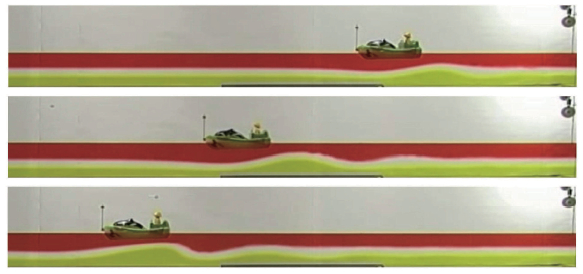Fig.1   Ekman’s dead water experiments reproduced with modern technology by French experimentalists in the laboratory of physics at of Lyon. The three-layer fluid system was used as the model(distinguished by red, white and yellow from top to bottom). The of each layer was 5.0 cm, 3.0 cm, and 5.5 cm respectively, and the density was 0.9967 g/cm$^3$, 1.0079 g/cm$^3$, and 1.0201 g/cm$^3$. The model ship sails from left to right, and snapshots from top to bottom show the position of the model as well as the internal wave profiles at different times

### 图2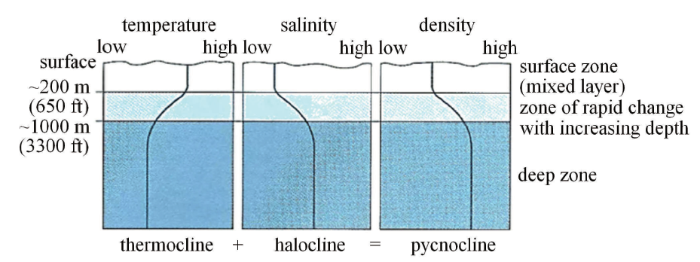Fig.2   Temperature, salinity, and density in the ocean as function of depth

### 图3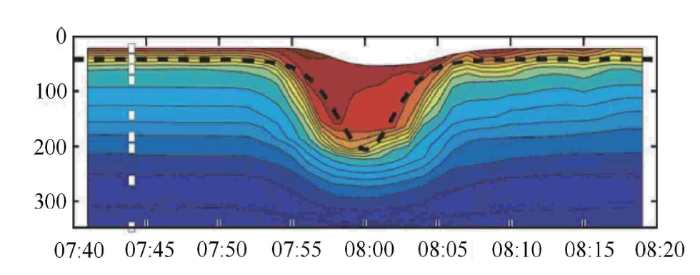Fig.3   A large-amplitude internal solitary wave observed in the South China Sea. The horizontal coordinate represents the time, sampling every 1 min, and the vertical coordinate indicates the depth of the ocean (the total depth is about 340 m)

### 图4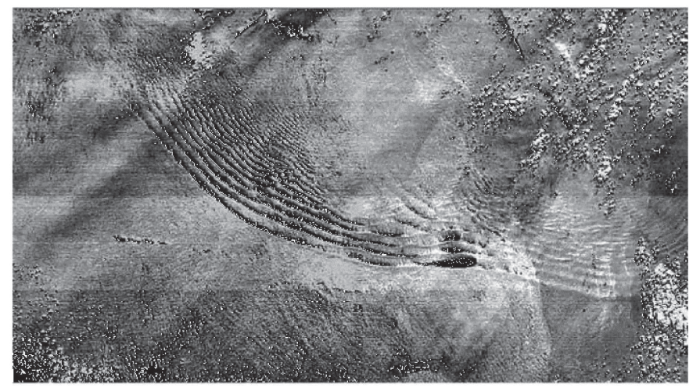Fig.4   SAR image of an internal solitary wave in the northern Dongsha islands (21 degrees north latitude, 116 degrees and 51 minutes east longitude)

### 图5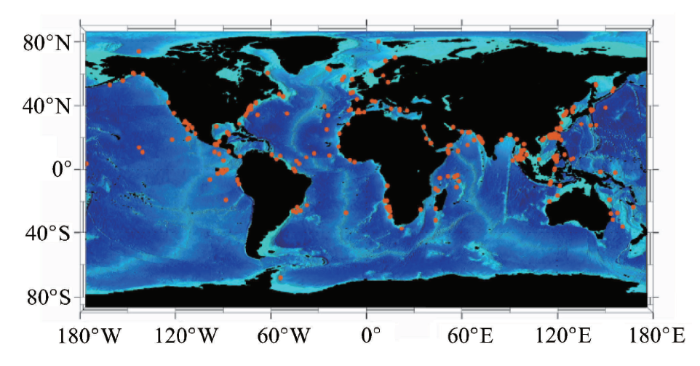Fig.5   Global distribution of internal solitary waves. Most of the locations were obtained by satellite remote sensing

## 1 密度强分层模型

20世纪70年代海洋卫星高空遥感技术的发展与应用成为人类认识海洋动力学的新起点. 对于海洋表面波与内波的研究成为了海洋工程、卫星遥感、水动力学、应用数学的汇聚之处. 随着人类对海洋探索的不断深入以及海洋工程的迫切需求, 近年来对于内波的理论与计算研究均取得了很大的进展, 许多非线性数学模型被构造出来, 而机理性的研究也朝着精细化和数学化的方向发展.

### 图6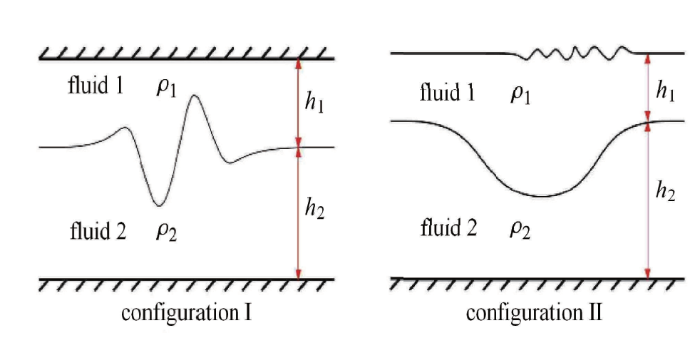Fig.6   Left panel: interfacial waves under the rigid-lid approximation. Right panel: interfacial waves with a free surface

1.1.1 弱非线性模型

$\begin{eqnarray} \eta_t+c\eta_x+\alpha_1\eta \eta_x+\beta_1\eta_{xxx}=0 \end{eqnarray}$

$\begin{eqnarray*} &&c = \left[\dfrac{g(\rho_2-\rho_1)h_2h_1}{\rho_2h_1+\rho_1h_2}\right]^{1/2}\\[1.5mm]&& \alpha_1 =\dfrac{3}{2}\dfrac{c}{h_1h_2}\dfrac{\rho_2h_1^2-\rho_1h_2^2}{\rho_2h_1+\rho_1h_2} \\[1.5mm]&& \beta_1 = \dfrac{ch_1h_2}{6}\dfrac{\rho_1h_1+\rho_2h_2}{\rho_2h_1+\rho_1h_2} \end{eqnarray*}$

$\begin{eqnarray} \omega=ck -\beta_1 k^3 \end{eqnarray}$

$\begin{eqnarray} \eta_t+c\eta_x+\alpha_1\eta \eta_x+\dfrac{\partial }{\partial x} \int^{+\infty}_{-\infty} G(x-x’)\eta(x’,t){\rm d}x’=0 \end{eqnarray}$

$G(\xi)=\dfrac {c}{2\pi} \int^{+\infty}_{-\infty} \lt[1-\dfrac{kh_1}{2} ({\rm coth} (kh_2)-1/kh_2) ] {\rm e}^{{\rm i}k\xi} {\rm d}k$

$c=\lt( \dfrac{\rho_2-\rho_1}{\rho_1} gh_1 )^{1/2}, \ \ \alpha_1=-\dfrac {3c}{2h_1}$

$\begin{eqnarray} \omega = ck\left[1-\frac{kh_1}{2}(\coth kh_2 - 1/kh_2)\right] \end{eqnarray}$

$\begin{eqnarray} \eta_t + c\eta_x + \alpha_1\eta \eta_x + \dfrac{\beta_1}{\pi}\dfrac{\partial^2}{\partial x^2} \int_{-\infty}^{+\infty} \dfrac{\eta(x’,t)}{x-x’} {\rm d}x’ = 0 \end{eqnarray}$

$\alpha_1 = -\frac{3c}{2h_1},\ \ \beta_1 = \frac{ch_1\rho_2}{2\rho_1}$

$\begin{eqnarray} \omega = ck - \beta_1k|k| \end{eqnarray}$

$\begin{eqnarray} \eta_t + \left(c+\alpha_1\eta+\alpha_2\eta^2\right)\eta_x + \beta_1\eta_{xxx} = 0 \end{eqnarray}$

1.1.2 强非线性模型

$\begin{eqnarray} && {\eta_1}_t + (\eta_1\bar{u}_1)_x = 0, \ \ \eta_1 = h_1-\eta\\ \end{eqnarray}$
$\begin{eqnarray} && {\eta_2}_t + (\eta_2\bar{u}_2)_x = 0, \ \ \eta_2 = h_2-\eta\\ \end{eqnarray}$
$\begin{eqnarray} && {\bar{u}_{1t}} + \bar{u}_1\bar{u}_{1x} + g\eta_x = \dfrac{P_x}{\rho_1} + \dfrac{1}{\eta_1}\left(\dfrac{1}{3}\eta_1^3G_1\right)_x + O\left(\beta^4\right)\qquad\\ \end{eqnarray}$
$\begin{eqnarray} && {\bar{u}_{2t}} + \bar{u}_2\bar{u}_{2x} + g\eta_x = \dfrac{P_x}{\rho_2} + \dfrac{1}{\eta_2}\left(\dfrac{1}{3}\eta_2^3G_2\right)_x + O\left(\beta^4\right) \end{eqnarray}$

$\begin{eqnarray} && \bar{u}_1(x,t) = \dfrac{1}{\eta_1}\int_{\eta}^{h_1}u_1(x,z,t) {\rm d}z\\ \end{eqnarray}$
$\begin{eqnarray}&& G_1(x,t) = \bar{u}_{1xt} + \bar{u}_1\bar{u}_{1xx} - (\bar{u}_{1x})^2 \end{eqnarray}$

$\bar{u}_2(x,t)$以及$G_2(x,t)$ 的定义类似, $P(x,t)$表示界面处的压强. 方程(8)是Choi和 Camassa在浅水假设下推导出来的演化方程, 在弱非线性假设下($\alpha = O(\beta^2)$), 可进一步导出KdV方程.

$\begin{eqnarray} \eta_t + c(\eta)\eta_x + (\beta(\eta)\eta_{xx})_x = 0 \end{eqnarray}$

$\beta(\eta) = \frac{1}{6}c(\eta)(h_1+\eta)(h_2-\eta)$

1.1.3 完全非线性的位势方程

(1) 流体的速度位势在各自区域内满足Laplace方程: $\Delta\phi_{1}=\Delta\phi_{2}=0$;

(2) 流体在上下固壁上满足无穿透边界条件: $\phi_{1z}=\phi_{2z}=0$;

(3) 在两层流体交界面$z=\eta(x,t)$上满足运动学边界条件(kinematic boundary condition)与动力学边界条件(dynamics boundary condition), 即

$\begin{eqnarray} &&\eta_t=\phi_{1z}-\eta_x\phi_{1x}=\phi_{2z}-\eta_x\phi_{2x}\\ \end{eqnarray}$
$\begin{eqnarray}&&\rho_1\left(\phi_{1t}+\dfrac12|\nabla\phi_1|^2+g\eta\right)-\\&&\qquad\rho_2\left(\phi_{2t}+\dfrac12|\nabla\phi_2|^2+g\eta\right)=0 \end{eqnarray}$

Turner和 Vanden-Broeck利用速度图变换和边界积分法对大振幅的内孤立波进行了数值计算, 他们发现随着振幅的增大内孤立波的脉冲部分变得越来越平、越来越宽, 最终演变成共轭流(conjugate flow). Laget和Dias利用相同的数值方法进行了计算, 并将结果与Koop和 Butler的实验数据进行了比较. Kataoka采用渐近分析技巧与数值谱分析法, 对内孤立波进行了纵向(二维扰动)和横向(三维扰动)的稳定性分析, 得到的基本结论是: 随着振幅的增大内孤立波出现不稳定, 但横向不稳定出现在纵向不稳定之前.

### 图7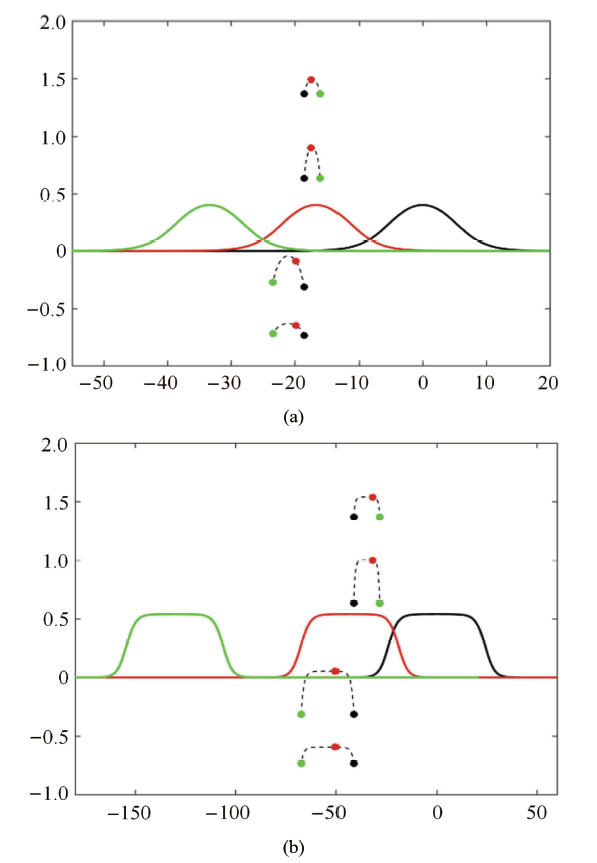Fig.7   Particle trajectories under internal solitary waves. Internal solitary waves move from left to right labeled with black, red, and green in order, and particle positions are marked as the corresponding colors. Numerical experiments were carried out in the fully nonlinear irrotational equations with the density ratio of 0.9 and depth ratio of 2

1.1.4 带自由面的二流体系统

$\begin{eqnarray} &&\widetilde{\eta}_t=\phi_{1z}-\widetilde{\eta}_{x}\phi_{1x} \\ \end{eqnarray}$
$\begin{eqnarray}&&\phi_{1t}+\dfrac12|\nabla\phi_1|^2+g\widetilde{\eta}=0 \end{eqnarray}$

### 图8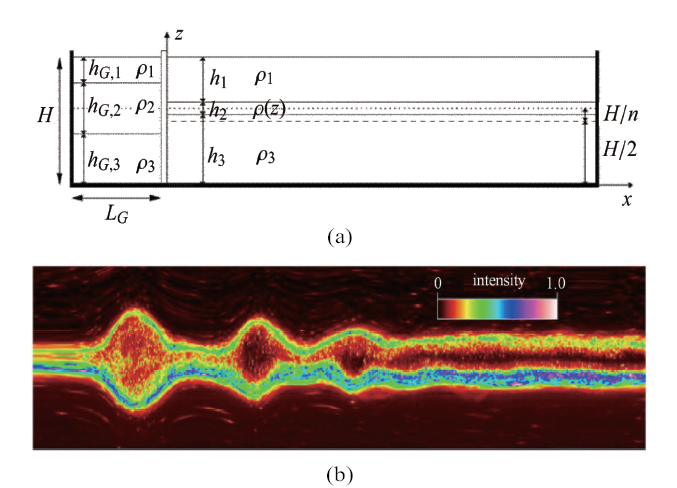Fig.8   Experimental setup for three-layer internal waves (top); group of mode-2 internal solitary waves (bottom). See Ref. for more details

## 2 连续密度分层

### 2.1 密跃层整体的脉动

2.1.1 弱非线性理论

$\begin{eqnarray}&& \dfrac{\partial }{\partial t} \psi_{zz} - b_x = \varepsilon J(\psi, \psi_{zz})-\mu\dfrac{\partial }{\partial t} \psi_{xx} + \varepsilon\mu J(\psi, \psi_{xx})\qquad\\ \end{eqnarray}$
$\begin{eqnarray}&& b_t + N^2(z)\psi_x = \varepsilon J(\psi, b) \end{eqnarray}$

$\begin{eqnarray*} N(z)=\sqrt{-\dfrac{g}{\rho_0} \dfrac{\partial \tilde \rho}{\partial z}} \end{eqnarray*}$

$\begin{eqnarray*} &&\psi = A(x,t)\phi(z) + \varepsilon A^2(x,t)c^2\phi^{(1,0)}(z) +\\&&\qquad\mu A_{xx}(x,t)c\phi^{(0,1)}(z) +\varepsilon^2A^3(x,t)\phi^{(2,0)}(z)+ \cdots\\ &&b = A(x,t)\frac{N^2(z)}{c}\phi(z) + \varepsilon A^2(x,t)D^{(1,0)}(z) + \\&&\qquad\mu A_{xx}(x,t)D^{(0,1)}(z) + \varepsilon^2A^3(x,t)D^{(2,0)}(z) + \cdots \end{eqnarray*}$

$\begin{eqnarray} B(x,t) = \dfrac{A(x,t)}{c} \end{eqnarray}$

$\begin{eqnarray} \mathcal{L}(\phi) = \phi''+\dfrac{N^2}{c^2}\phi = 0 \end{eqnarray}$

$\begin{eqnarray} \phi(0) =\phi(1) = 0 \end{eqnarray}$

$\begin{eqnarray} B_t + cB_x = 0 \end{eqnarray}$

$\begin{eqnarray} B_t + cB_x + \varepsilon\alpha BB_x + \mu\beta B_{xxx} = 0 \end{eqnarray}$

$\begin{eqnarray} B_t + cB_x + \varepsilon\alpha BB_x + \mu\beta B_{xxx}+ \varepsilon^2\alpha_1 B^2B_x = 0 \end{eqnarray}$

2.1.2 完全非线性理论

$\begin{eqnarray} \nabla ^2 \eta + \frac{N^2(z-\eta)}{c^2}\eta =0 \end{eqnarray}$

$\begin{eqnarray} \eta(x,0) = \eta(x,H) = 0 , \ \ \mathop{\lim}\limits_{x\to \pm \infty} \eta(x,z) = 0 \end{eqnarray}$

$\begin{eqnarray} &&\nabla ^2 \eta + \dfrac{N_{\rm NB}^2(z-\eta)}{c^2}\eta + \\&&\qquad\dfrac{N_{\rm NB}^2(z-\eta)}{2g} \left[\eta_x^2+\eta_z(\eta_z-2)\right] = 0 \end{eqnarray}$

### 2.2 密跃层内部的波动

2.2.1 圣$\cdot$安德鲁十字现象

### 图9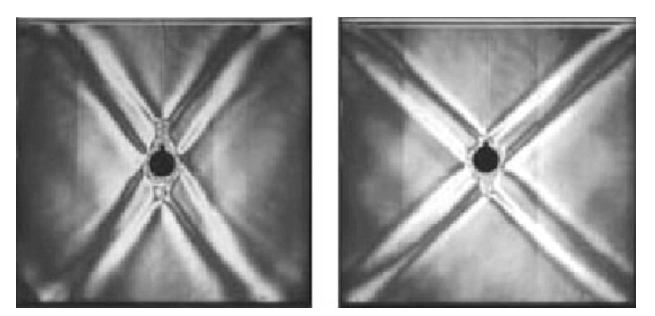Fig.9   Visualization of St. Andrew’s cross excited by a vertically oscillating circular cylinder in a density stratified fluid with constant buoyance frequency. The angle of arms is determined by the period (or frequency equivalently) of oscillation which is 5 s on the left and 6 s on the right (http://www.gfd-dennou.org/library/gfd_exp/exp_e/doc/iw/guide01.htm)

### 图10Fig.10   Contours of zonal velocity (upper panel) and vertical velocity (lower panel) superposed on temperature contours (dashed lines) and cloud contours (heavy solid lines) near the 12 km level

$\begin{eqnarray} \omega = N\sqrt{\dfrac{k_x^2+k_y^2}{k_x^2+k_y^2+k_z^2}} = N \cos\theta \end{eqnarray}$

$\begin{eqnarray*} &&{c}_p = \dfrac{\omega}{K}{e}_{K} = \dfrac{\omega}{K^2}(k_x, k_y, k_z)\\ &&{c}_g = \dfrac{{\rm d}\omega}{{\rm d} {K}}=\dfrac{Nk_z}{K^3\sqrt{k_x^2+k_y^2}} \lt[k_xk_z, k_yk_z, -(k_x^2+k_y^2)] \end{eqnarray*}$

### 图11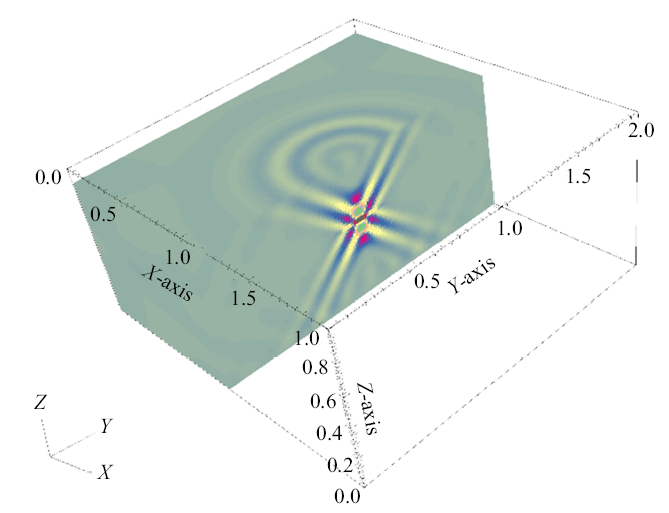Fig.11   DNS of St. Andrew’s cross in 3D Boussinesq equations based on a fourth-order (in both space and time) numerical scheme

2.2.2 圣$\cdot$安德鲁十字的理论与数值进展

$\begin{eqnarray} && \frac{\partial u}{\partial x} + \frac{\partial v}{\partial z} =0\\ \end{eqnarray}$
$\begin{eqnarray} && \frac{\partial u}{\partial t} + u\frac{\partial u}{\partial x} + v\frac{\partial u}{\partial z} = -\frac{p_x}{\rho_0}+\nu \Delta u\\ \end{eqnarray}$
$\begin{eqnarray}&& && \frac{\partial v}{\partial t} + u\frac{\partial v}{\partial x} + v\frac{\partial v}{\partial z} = -\frac{p_z}{\rho_0} - \frac{\rho g}{\rho_0} + \nu \Delta v + F\\ \end{eqnarray}$
$\begin{eqnarray}&& \frac{\partial \rho}{\partial t} + u\frac{\partial \rho}{\partial x} + v\frac{\partial \rho}{\partial z} + v\frac{\partial \widetilde{\rho}}{\partial z} = 0 \end{eqnarray}$

2.2.3 内波湍流与潜艇隐身

### 图12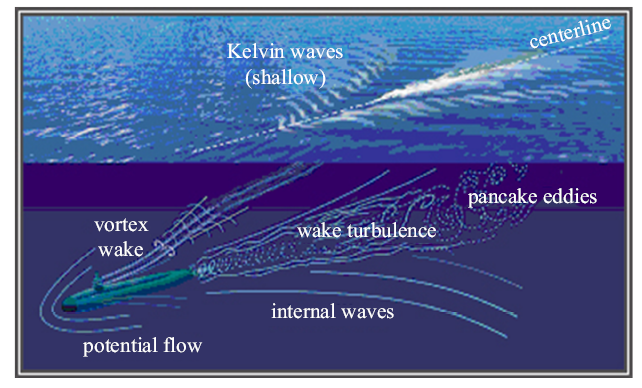Fig.12   Various wakes generated by motion of submarine: Kelvin wake, internal waves, wake turbulence, vortex wake, and pancake eddies. The figure comes from the internet

## 3 展 望

### 3.1 内波中的多界面耦合问题

(1) 内波与海洋表面波的相互作用: 大振幅内孤立波诱发海洋表面的激潮现象, 为使用卫星遥感图片反演内孤立波提供了途径, 具有重要的应用价值; 但这一问题的多尺度效应极为明显, 以早期印度洋东部安达曼海实测数据为例, Perry 和Schimke观测到南北(波脊线方向)长30 km、东西宽200$\sim$800 m的波浪区, 这样的浪区至少有5个, 间隔约为3200 m, 其中的波浪是高为0.3$\sim$0.6 m、波峰很尖的短波, 他们通过测量仪器辨明了浪区下有振幅达80 m、长约2 km的内孤立波通过; 这里的尺度仅在空间上来讲就需要考虑米量级(表面短波)、百米量级(表面波波包)、千米量级(内波)等; 目前一般使用射线理论(Ray theory)来解释“激潮现象”(见文献[47-48, 102]等), 但仍存在一定的争议; 因此构造新的多尺度模型或者发展高精度的计算方法用于理解内孤立波激发海洋表面波群的机理, 以及确定两个自由面间的定量关系是当务之急.

(2) 内波高阶模态的性质: 内波的高阶模态一般无法用“两层模型+刚盖假设”来描述, 但当外在条件(如海底地形)变化时内波模态之间的相互转换具有重要的科学意义(见文献中的实验研究 和文献中的理论研究); 为深刻理解这其中的关键, 发展三层乃至更多层的内波模型研究多界面耦合问题是一个有意义的方向.

### 图13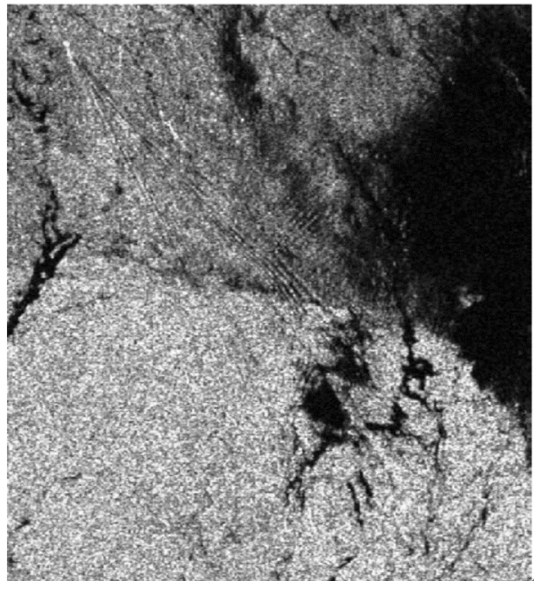Fig.13   SAR image of a Kelvin wake in the strait of Georgia

### 图14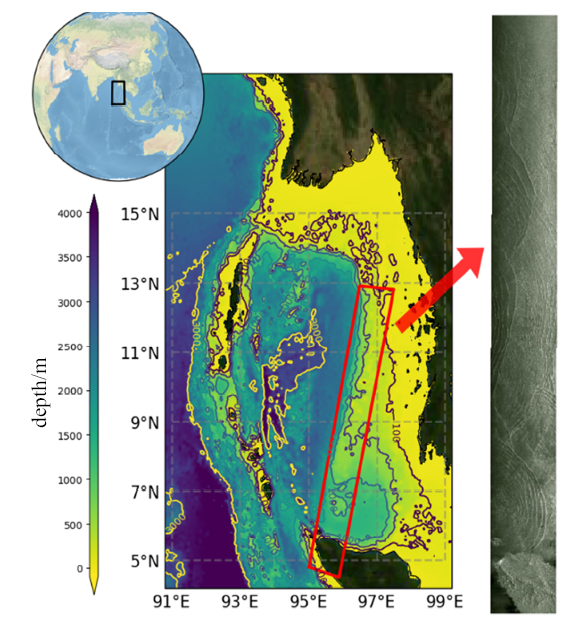Fig.14   Bathymetric map of part of the Andaman Sea (left panel). SAR image taken by ERS-2 satellite in the Andaman Sea on February 11, 1997 (right panel) shows wave-wave interactions of internal solitary waves. This figure is provided by Dr. Yuan from Ocean University of China

### 图15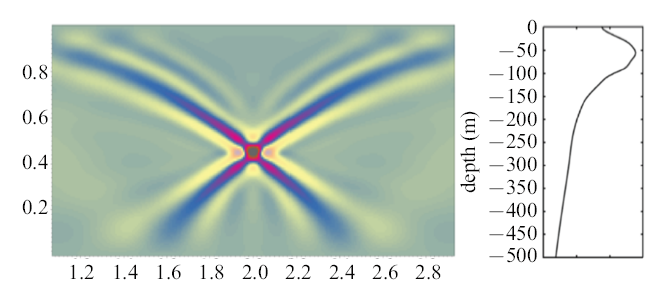Fig.15   St. Andrew’s cross in the South China Sea. The fourth-order numerical scheme was used to carry out the numerical experiment in the Boussinesq equations with the Reynolds number of $2.5\times 10^{4}$

## 参考文献 原文顺序 文献年度倒序 文中引用次数倒序 被引期刊影响因子

Mercier MJ, Vasseur R, Dauxois T .

Nonlinear Processes in Geophysics, 2011,18:193-208

Perry RB, Schimke GR .

Large-amplitude internal waves observed off the northwest coast of Sumatra

Journal of Geophysical Research, 1965,70(10):2319-2324

Osborne AR, Burch TL .

Internal solitons in the Andaman Sea

Science, 1980,208(4443):451-460

URL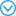The solitary wave is a localized hydrodynamic phenomenon that can occur because of a balance between nonlinear cohesive and linear dispersive forces in a fluid. It has been shown theoretically, and observed experimentally, that some solitary waves have properties analogous to those of elementary particles, and the waves have therefore been named solitons. During a measurement program in the Andaman Sea near northern Sumatra, large-amplitude, long internal waves were observed with associated surface waves called tide rips. Using theoretical results from the physics of nonlinear waves, it is shown that the internal waves are solitons and their interactions with surface waves are described.

Duda TF, Lynch JF, Irish JD , et al.

Internal tide and nonlinear internal wave behavior at the continental slope in the northern South China Sea

IEEE Journal of Oceanic Engineering, 2005,29(4):1105-1130

5种劲松, 周晓中 .

URL( Chong Jinsong, Zhou Xiaozhong .

Survey of study on internal waves detection in synthetic aperture radar image

Journal of Radars, 2013,2(4):406-421 (in Chinese))

URLCaponi EA, Crawford DR, Yuen HC , et al.

Modulation of radar backscatter from the ocean by a variable surface current

Journal of Geophysical Research Oceans, 1988,93(C10):12249-12263

Alpers W .

Theory of radar imaging of internal waves

Nature, 1985,314(6008):245-247

Jackson C .

Internal wave detection using the moderate resolution imaging spectroradiometer (modis)

Journal of Geophysical Research: Oceans, 2007,112:C11012

( Li Jiachun .

Billow under the sea surface-Internal waves in the ocean

Mechanics in Engineering, 2005,27(2):3-8 (in Chinese))

URL( Cai Shuqun, He Jianling, Xie Jieshuo .

Recent decadal progress of the study on internal solitons in the South China Sea

Advances in Earth Science, 2011,26(7):703-710 (in Chinese))

URLURL( Cai Shuqun, Gan Zijun .

Progress in the study of the internal soliton in the northern South China Sea

Advances in Earth Science, 2001,16(2):215-219 (in Chinese))

URLApel JR, Ostrovsky LA, Stepanyants YA , et al.

Internal solitons in the ocean and their effect on underwater sound

The Journal of the Acoustical Society of America, 2007,121:695-722

URLNonlinear internal waves in the ocean are discussed (a) from the standpoint of soliton theory and (b) from the viewpoint of experimental measurements. First, theoretical models for internal solitary waves in the ocean are briefly described. Various nonlinear analytical solutions are treated, commencing with the well-known Boussinesq and Korteweg-de Vries equations. Then certain generalizations are considered, including effects of cubic nonlinearity, Earth's rotation, cylindrical divergence, dissipation, shear flows, and others. Recent theoretical models for strongly nonlinear internal waves are outlined. Second, examples of experimental evidence for the existence of solitons in the upper ocean are presented; the data include radar and optical images and in situ measurements of wave forms, propagation speeds, and dispersion characteristics. Third, and finally, action of internal solitons on sound wave propagation is discussed. This review paper is intended for researchers from diverse backgrounds, including acousticians, who may not be familiar in detail with soliton theory. Thus, it includes an outline of the basics of soliton theory. At the same time, recent theoretical and observational results are described which can also make this review useful for mainstream oceanographers and theoreticians.

Joseph RI .

Solitary waves in a finite depth fluid

Journal of Physics A General Physics, 1977,10(12):L225-L227

URLGeneralized solitary waves propagating at the surface of a fluid of finite depth are considered. The fluid is assumed to be inviscid and incompressible and the flow to be irrotational. Both the effects of gravity and surface tension are included. It is shown that in addition to the classical symmetric waves, there are new asymmetric solutions. These new branches of solutions bifurcate from the branches of symmetric waves. The detailed bifurcation diagrams as well as typical wave profiles are presented.

Kubota T .

Weakly-nonlinear, long internal gravity waves in stratified fluids of finite depth

Journal of Hydronautics, 1978,12(4):157-165

Benjamin, Brooke T .

Internal waves of permanent form in fluids of great depth

Journal of Fluid Mechanics, 1967,29(3):559-592

Davis RE, Acrivos A .

Solitary internal waves in deep water

Journal of Fluid Mechanics, 2006,29(3):593-607

URLInternal solitary waves (ISWs) can cause strong vertical and horizontal currents and turbulent mixing in the ocean. These processes affect sediment and pollutant transport, acoustic transmissions and man-made structures in the shallow and deep oceans. Previous studies of the role of ISWs in suspending seafloor sediments and forming marine nepheloid layers were mainly conducted in shallow-water environments. In summer 2017, we observed at least four thick (70-140 m) benthic nepheloid layers (BNLs) at water depths between 956 and 1545 m over continental slopes in the northern South China Sea. We found there was a good correlation between the timing of the ISW packet and variations of the deepwater suspended sediment concentration (SSC). At a depth of 956 m, when the ISW arrived, the near-bottom SSC rapidly increased by two orders of magnitude to 0.62 mg/l at 8 m above the bottom. At two much deeper stations, the ISW-induced horizontal velocity reached 59.6-79.3 cm/s, which was one order of magnitude more than the seafloor contour currents velocity. The SSC, 10 m above the sea floor, rapidly increased to 0.10 mg/l (depth of 1545 m) and 1.25 mg/l (depth of 1252 m). In this study, we found that ISWs could suspend much more sediments on deepwater areas than previously thought. Specifically, we estimated that ISWs could induce and suspend 78.7 Mt/yr of sediment from shelf to deep-sea areas of the northern South China Sea. The total amount of sediment resuspended by shoaling ISWs was 2.7 times that of river-derived sediment reaching the northern South China Sea. This accounted for 6.1% of the global river-discharged sediment (16.4% of that from Asian rivers) transported to the sea.

Ono H .

Algebraic solitary waves in stratified fluids

Journal of the Physical Society of Japan, 1975,39(4):1082-1091

Matsuno Y .

A unified theory of nonlinear wave propagation in two-layer fluid systems

Journal of the Physical Society of Japan, 2007,62(6):1902-1916

Choi W, Camassa R .

Weakly nonlinear internal waves in a two-fluid system

Journal of Fluid Mechanics, 1996,313:83-103

Grue J, Jensen A ,

Rus$\mathring{a}$s PO. Properties of large-amplitude internal waves

Journal of Fluid Mechanics, 2000,380:257-278

Koop CG, Butler G .

An investigation of internal solitary waves in a two-fluid system

Journal of Fluid Mechanics, 1981,112:225-251

Michallet H ,

Barth$\acute{e}$lemy E. Experimental study of interfacial solitary waves

Journal of Fluid Mechanics, 1998,366:159-177

URLWe propose consistent scaling of solitary waves on inertia-dominated falling liquid films, which accurately accounts for the driving physical mechanisms and leads to a self-similar characterization of solitary waves. Direct numerical simulations of the entire two-phase system are conducted using a state-of-the-art finite volume framework for interfacial flows in an open domain that was previously validated against experimental film-flow data with excellent agreement. We present a detailed analysis of the wave shape and the dispersion of solitary waves on 34 different water films with Reynolds numbers Re=20-120 and surface tension coefficients σ=0.0512-0.072 N m(-1) on substrates with inclination angles β=19°-90°. Following a detailed analysis of these cases we formulate a consistent characterization of the shape and dispersion of solitary waves, based on a newly proposed scaling derived from the Nusselt flat film solution, that unveils a self-similarity as well as the driving mechanism of solitary waves on gravity-driven liquid films. Our results demonstrate that the shape of solitary waves, i.e., height and asymmetry of the wave, is predominantly influenced by the balance of inertia and surface tension. Furthermore, we find that the dispersion of solitary waves on the inertia-dominated falling liquid films considered in this study is governed by nonlinear effects and only driven by inertia, with surface tension and gravity having a negligible influence.

Lee CY, Beardsley RC .

The generation of long nonlinear internal waves in a weakly stratified shear flow

Journal of Geophysical Research, 1974,79(3):453-462

Helfrich KR, Melville WK .

Long nonlinear internal waves

Annual Review of Fluid Mechanics, 2006,38:395-425

URLNonlinear internal waves in shallow water have significant acoustic impacts and cause three-dimensional ducting effects, for example, energy trapping in a duct between curved wavefronts that propagates over long distances. A normal mode approach applied to a three-dimensional idealized parametric model [Lin, McMahon, Lynch, and Siegmann, J. Acoust. Soc. Am. 133(1), 37-49 (2013)] determines the dependence of such effects on parameters of the features. Specifically, an extension of mode number conservation leads to convenient analytical formulas for along-duct (angular) acoustic wavenumbers. The radial modes are classified into five types depending on geometric characteristics, resulting in five distinct formulas to obtain wavenumber approximations. Examples of their dependence on wavefront curvature and duct width, along with benchmark comparisons, demonstrate approximation accuracy over a broad range of physical values, even including situations where transitions in mode types occur with parameter changes. Horizontal-mode transmission loss contours found from approximate and numerically exact wavenumbers agree well in structure and location of intensity features. Cross-sectional plots show only small differences between pattern phases and amplitudes of the two calculations. The efficiency and accuracy of acoustic wavenumber and field approximations, in combination with the mode-type classifications, suggest their application to determining parameter sensitivity and also to other feature models.

Miyata M .

Long internal waves of large amplitude

IUTAM Symposinm of Nonlinear Water Waves, Tokyo, Janpan, Aug. 25-28, 1987-1988

URLThe Fourth Universal Definition of Myocardial Infarction defines electrocardiographic (ECG) Q waves as duration ≥30ms and amplitude ≥1mm or QS complex in two contiguous leads. However, current taskforce criteria may be overly restrictive. Therefore, we investigated the association of isolated, lenient, or strict Q waves with long-term outcome.

Choi W, Camassa R .

Fully nonlinear internal waves in a two-fluid system

Journal of Fluid Mechanics, 1999,396:1-36

Jo TC, Choi W .

Dynamics of strongly nonlinear internal solitary waves in shallow water

Studies in Applied Mathematics, 2010,109(3):205-227

Ostrovsky Lev A, Grue J .

Evolution equations for strongly nonlinear internal waves

Physics of Fluids, 2003,15(10):2934-2948

URLUsing an integrable Gardner equation as an example, a perturbation theory is developed for systems in which limiting-amplitude solitons exist in the form of a pair of distanced kinks. Approximate equations describing multisoliton interactions are derived and further used for modeling the evolution of an arbitrary set of solitons. The results are compared with an exact solution and numerical results. The theory is applied to data from observation of a train of strongly nonlinear internal waves in the ocean.

Turner REL, Vanden-Broeck JM .

Physics of Fluids, 1988,31(9):2486-2490

URLSteady solitary and generalized solitary waves of a two-fluid problem where the upper layer is under a flexible elastic sheet are considered as a model for internal waves under an ice-covered ocean. The fluid consists of two layers of constant densities, separated by an interface. The elastic sheet resists bending forces and is mathematically described by a fully nonlinear thin shell model. Fully localized solitary waves are computed via a boundary integral method. Progression along the various branches of solutions shows that barotropic (i.e. surface modes) wave-packet solitary wave branches end with the free surface approaching the interface. On the other hand, the limiting configurations of long baroclinic (i.e. internal) solitary waves are characterized by an infinite broadening in the horizontal direction. Baroclinic wave-packet modes also exist for a large range of amplitudes and generalized solitary waves are computed in a case of a long internal mode in resonance with surface modes. In contrast to the pure gravity case (i.e without an elastic cover), these generalized solitary waves exhibit new Wilton-ripple-like periodic trains in the far field.

Laget O, Dias F .

Numerical computation of capillary-gravity interfacial solitary waves

Journal of Fluid Mechanics, 1997,349:221-251

Kataoka T .

The stability of finite-amplitude interfacial solitary waves

Fluid Dynamics Research, 2006,38(12):831-867

URL## Abstract

The linear stability of finite-amplitude interfacial gravity solitary waves propagating in a two-layer fluid is investigated analytically focusing on the occurrence of an exchange of stability. We make an asymptotic analysis for small growth rates of infinitesimal disturbances, and explicitly obtain their growth rates near an exchange of stability. The result indicates that an exchange of stability occurs at every stationary value of the total energy of the solitary waves. It also gives us information whether the number of growing modes increases or decreases after experiencing the exchange of stability. We apply these analytical results to specific interfacial solitary waves, and find various features on their stability that are not seen in the case of surface solitary waves.

Vanden-Broeck J-M .

Numerical calculation of gravity-capillary interfaical waves of finite amplitude

Physics of Fluids, 1980,23:1723-1726

Turner REL, Vanden-Broeck J-M .

The limiting configuration of interfacial gravity waves

Physics of Fluids, 1986,29:372-375

Dias F, Vanden-Broeck J-M .

On internal fronts

Journal of Fluid Mechanics, 2003,479:145-154

URLEpitaxial lateral overgrowth (ELO) over a free-standing dielectric mask is an unexplored territory in selective epitaxy growth (SEG) of semiconductors. By shrinking the dielectric mask dimension to the micron scale, the growth fronts from ELO are able to converge and coalesce, thus providing the freedom to engineer the interfacial structure between the epi-layer and dielectric mask. We demonstrate, herein, anomalous adatom diffusion and migration at the Ge/SiO2 interface upon SEG on a Si (100) wafer. We find, depending on the oxide strip length, a polyhedral cavity or tunnel can form on the oxide layer. More importantly, we observe a thermally induced substantial internal surface reconfiguration process of Ge atoms that connects two tunnels and one cavity in order to form a single tunnel. Defect-free Ge above the oxide strips is obtained after coalescence. Our findings yield new insight into adatom migration in an enclosed space, and the cavity and tunnel show the first known three-dimensional geometric configuration in selective heteroepitaxial structures.

Sha H, Vanden-Broeck JM .

Two-layer flows past a semicircular obstruction

Physics of Fluids, 1993,5:2661-2668

Dias F, Vanden-Broeck JM .

Steady two-layer flows over an obstacle

Philosophical Transactions of the Royal Society A, 2002,360:2137-2154

URLNonlinear waves in a forced channel flow of two contiguous homogeneous fluids of different densities are considered. Each fluid layer is of finite depth. The forcing is due to an obstruction lying on the bottom. The study is restricted to steady flows. First a weakly nonlinear analysis is performed. At leading order the problem reduces to a forced Korteweg-de Vries equation, except near a critical value of the ratio of layer depths which leads to the vanishing of the nonlinear term. The weakly nonlinear results obtained by integrating the forced Korteweg-de Vries equation are validated by comparison with numerical results obtained by solving the full governing equations. The numerical method is based on boundary integral equation techniques. Although the problem of two-layer flows over an obstacle is a classical problem, several branches of solutions which have never been computed before are obtained.

Dias F, Vanden-Broeck JM .

Two-layer hydraulic falls over an obstacle

European Journal of Mechanics B/Fluids, 2004,23:879-898

Hou TY, John SL, Michael JS .

Removing the stiffness from interfacial flows with surface tension

Journal of Computational Physics, 1994,114(2):312-338

Meiron DI, Saffman PG .

Oerhanging interfacial gravity waves of large amplitude

Journal of Fluid Mechanics, 1983,129:213-218

URLInternal solitary waves are hump-shaped, large-amplitude waves that are physically analogous to surface waves except that they propagate within the fluid, along density steps that typically characterize the layered vertical structure of lakes, oceans and the atmosphere. As do surface waves, internal solitary waves may overturn and break, and the process is thought to provide a globally significant source of turbulent mixing and energy dissipation. Although commonly observed in geophysical fluids, the origins of internal solitary waves remain unclear. Here we report a rarely observed natural case of the birth of internal solitary waves from a frontally forced interfacial gravity current intruding into a two-layer and vertically sheared background environment. The results of the analysis carried out suggest that fronts may represent additional and unexpected sources of internal solitary waves in regions of lakes, oceans and atmospheres that are dynamically similar to the situation examined here in the Saguenay Fjord, Canada.

Grimshaw RHJ, Pullin DI .

Extreme interfacial waves

Physics of Fluids, 1986,29:2802-2807

Akers B, Ambrose DM, Wright JD .

Traveling waves from the arclength parameterization: Vortex sheets with surface tension

Interfaces and Free Boundaries, 2013,15:359-380

URLWe study traveling waves for the vortex sheet with surface tension. We use the angle-arclength description of the interface rather than Cartesian coordinates, and we utilize an arclength parameterization as well. In this setting, we make a new formulation of the traveling wave ansatz. For this problem, it should be possible for traveling waves to overturn, and notably, our formulation does allow for waves with multi-valued height. We prove that there exist traveling vortex sheets with surface tension bifurcating from equilibrium. We compute these waves by means of a quasi-Newton iteration in Fourier space; we find continua of traveling waves bifurcating from equilibrium and extending to include overturning waves, for a variety of values of the mean vortex sheet strength.

Wilkening J .

Breakdown of self-similarity at the crests of large amplitude standing water waves

Physical Review Letters, 2011,107:184501

URLWe study the limiting behavior of large-amplitude standing waves on deep water using high-resolution numerical simulations in double and quadruple precision. While periodic traveling waves approach Stokes's sharply crested extreme wave in an asymptotically self-similar manner, we find that standing waves behave differently. Instead of sharpening to a corner or cusp as previously conjectured, the crest tip develops a variety of oscillatory structures. This causes the bifurcation curve that parametrizes these waves to fragment into disjoint branches corresponding to the different oscillation patterns that occur. In many cases, a vertical jet of fluid pushes these structures upward, leading to wave profiles commonly seen in wave tank experiments. Thus, we observe a rich array of dynamic behavior at small length scales in a regime previously thought to be self-similar.

Ambrose DM, Wilkening J .

Computation of symmetric, time-periodic solutions of the vortex sheet with surface tension

Proceedings of the National Academy of Sciences of the United States of America, 2010,108(8):3361-3366

URLA numerical method is introduced for the computation of time-periodic vortex sheets with surface tension separating two immiscible, irrotational, two-dimensional ideal fluids of equal density. The approach is based on minimizing a nonlinear functional of the initial conditions and supposed period that is positive unless the solution is periodic, in which case it is zero. An adjoint-based optimal control technique is used to efficiently compute the gradient of this functional. Special care is required to handle singular integrals in the adjoint formulation. Starting with a solution of the linearized problem about the flat rest state, a family of smooth, symmetric breathers is found that, at quarter-period time intervals, alternately pass through a flat state of maximal kinetic energy, and a rest state in which all the energy is stored as potential energy in the interface. In some cases, the interface overturns before returning to the initial, flat configuration. It is found that the bifurcation diagram describing these solutions contains several disjoint curves separated by near-bifurcation events.

Wang Z, Guan X .

Mass transport by fully nonlinear internal waves in two-layer fluids

Preprint, 2019

Kodaira T, Waseda T, Miyata M , et al.

Internal solitary waves in a two-fluid system with a free surface

Journal of Fluid Mechanics, 2016,804:201-223

Forgia G. la, Sciortino G .

The role of the free surface on interfacial solitary waves

Physics of Fluids, 2019,31:106601

Craig W, Guyenne D, Kalisch H .

Communication on Pure and Applied Mathematics, 2005,58:1587-1641

Haut TS, Ablowitz MJ .

A reformulation and applications of interfacial fluids with a free surface

Journal of Fluid Mechanics, 2009,631:375-396

Barros R, Gavrilyuk SL, Teshukov VM .

Dispersive nonlinear waves in two-layer flows with free surface. i. Model derivation and general properties

Studies in Applied Mathematics, 2007,119(3):191-211

Fochesato C, Dias F, Grimshaw R .

Generalized solitary waves and fronts in coupled korteweg-de vries systems

Physica D, 2005,210:96-117

Dias F, Il’ichev A .

Interfacial waves with free-surface boundary conditions: An approach via a model equation

Physica D, 2001,150:278-300

Moni JN, King AC .

Guided and unguided interfacial solitary waves

Quarterly Journal of Mechanics and Applied Mathematics, 1995,48:21-38

Michallet H, Dias F .

Numerical study of generalized interfacial solitary waves

Physics of Fluids, 1999,11(6):1502-1511

URLSteady solitary and generalized solitary waves of a two-fluid problem where the upper layer is under a flexible elastic sheet are considered as a model for internal waves under an ice-covered ocean. The fluid consists of two layers of constant densities, separated by an interface. The elastic sheet resists bending forces and is mathematically described by a fully nonlinear thin shell model. Fully localized solitary waves are computed via a boundary integral method. Progression along the various branches of solutions shows that barotropic (i.e. surface modes) wave-packet solitary wave branches end with the free surface approaching the interface. On the other hand, the limiting configurations of long baroclinic (i.e. internal) solitary waves are characterized by an infinite broadening in the horizontal direction. Baroclinic wave-packet modes also exist for a large range of amplitudes and generalized solitary waves are computed in a case of a long internal mode in resonance with surface modes. In contrast to the pure gravity case (i.e without an elastic cover), these generalized solitary waves exhibit new Wilton-ripple-like periodic trains in the far field.

P$\breve{a}$r$\breve{a}$u EI, Dias F .

Interfacial periodic waves of permanent form with free-surface boundary conditions

Journal of Fluid Mechanics, 2001,437:325-336

Wang Z, P$\breve{a}$r$\breve{a}$u EI, Milewski PA , et al.

Numerical study of interfacial solitary waves propagating under an elastic sheet

Proceedings of the Royal Society A, 2014,470(2168):20140111

URLSteady solitary and generalized solitary waves of a two-fluid problem where the upper layer is under a flexible elastic sheet are considered as a model for internal waves under an ice-covered ocean. The fluid consists of two layers of constant densities, separated by an interface. The elastic sheet resists bending forces and is mathematically described by a fully nonlinear thin shell model. Fully localized solitary waves are computed via a boundary integral method. Progression along the various branches of solutions shows that barotropic (i.e. surface modes) wave-packet solitary wave branches end with the free surface approaching the interface. On the other hand, the limiting configurations of long baroclinic (i.e. internal) solitary waves are characterized by an infinite broadening in the horizontal direction. Baroclinic wave-packet modes also exist for a large range of amplitudes and generalized solitary waves are computed in a case of a long internal mode in resonance with surface modes. In contrast to the pure gravity case (i.e without an elastic cover), these generalized solitary waves exhibit new Wilton-ripple-like periodic trains in the far field.

Rusas P-O, Grue J .

Solitary waves and conjugate flows in a three-layer fluid

European Journal of Mechanics B/Fluids, 2002,21:185-206

Fructus D, Grue J .

Fully nonlinear solitary waves in a layered stratified fluid

Journal of Fluid Mechanics, 2004,505:323-347

Vanden-Broeck JM, Turner REL .

Long periodic internal waves

Physics of Fluids, 1992,4:1929-1935

URLHypokalemic periodic paralysis (HOKPP) is a rare neuromuscular disorder caused by altered transport of cellular potassium that leads to significant muscle weakness of the extremities. Paralytic attacks are induced by a drop in the serum potassium level and they have been associated with specific triggers. This case describes a 21-year-old male who has had recurrent presentations of acute paralytic attacks following vigorous physical activity. At presentation, this patient exhibited flaccid paralysis of all skeletal muscles below the neck, but was alert and oriented with stable vital signs. The patient was found to have a potassium level of 2.1 mmol/L and an EKG demonstrating U waves (characteristic of hypokalemia). The patient was treated with potassium supplementation with resolution of symptoms. The mainstay of prevention of long term permanent muscle weakness is avoidance of triggers that can lead to hypokalemia. Through education on disease process and lifestyle modifications, we were able to end the cycle of recurrent hospital readmissions and the subsequent financial burden this generated for the patient and his family.

Sha H, Vanden-Broeck JM .

Internal solitary waves with stratification in density

Journal of the Australian Mathematical Society Series B, 1997,38:563-580

Carr M, Davies PA, Hoebers RP .

Experiments on the structure andstability of mode-2 internal solitary-like waves propagating on an offset pycnocline

Physics of Fluids, 2015,27:046602

Deepwell D, Stastna M, Carr M , et al.

Interaction of a mode-2 internal solitary wave with narrow isolated topography

Physics of Fluids, 2017,29:076601

Carr M, Stastna M, Davies PA , et al.

Shoaling mode-2 internal solitary-like waves

Journal of Fluid Mechanics, 2019,879:604-632

Ambrose DM .

Well-posedness of vortex sheets with surface tension

SIAM Journal of Mathematical Analysis, 2003,35(1):211-244

Benney DJ .

Long non-linear waves in fluid flows

Journal of Mathematical Physics, 1966,45:52-63

Lee CY, Beardsley RC .

The generation of long nonlinear internal waves in a weakly stratified shear flow

Journal of Geophysical Research, 1974,79:453-462

Dubreil-Jacotin ML .

Sur la d$\acute{e}$termination rigoureuse des ondes permanentes p$\acute{e}$riodiques d’ampleur finie

[PhD Thesis]. Gauthier-Villars, 1934

Long RR .

Some aspects of the flow of stratified fluds I. A theoretical investigation

Tellus, 1953,5:42-58

URLTheoretical investigation is performed to explore the novel aspects of nonlinear thermal radiation and non-uniform heat source/sink for chemically reactive flow of ferromagnetic Maxwell liquid over a permeable stretching sheet. Buongiorno model is employed to include Brownian motion and thermophoresis effects. The novelty of the existing study is to account the effect of binary chemical reaction, viscous dissipation, thermal and solutal stratification for ferromagnetic Maxwell fluid. Governing system of nonlinear partial differential equations is transformed into a system of nonlinear ordinary differential equations with the help of apposite similarity transformations. The acquired resulting nonlinear ODEs are solved numerically with the assistance built-in-shooting method (bvp4c). Effects of emanating variables are examined through graphs and tables. It is evident that heat transfer rate enhances with thermal radiation. It is analyzed that temperature upsurges for greater estimations of thermal radiation $( N 1 ∗ )$ , ferromagnetic $( β ˆ 2 )$ and thermophoresis $( N ˆ t )$ parameters however it declines for Prandtl number (Pr) and thermal stratified parameter (S₁). Space and temperature dependent heat sinks are more appropriate for cooling purposes.

Stastna M, Lamb KG .

Large fully nonlinear internal solitary waves: The effect of background current

Physics of Fluids, 2002,14(9):2987-2999

Mowbray DE, Rarity BSH .

A theoretical and experimental investigation of the phase configuration of internal waves of small amplitude in a density stratified liquid

Journal of Fluid Mechanics, 1967,56(1):1-16

Lighthill MJ. Waves in Fluids. Cambridge University Press, 1978

Haynes PH, Marks CJ, Mclntyre ME , et al.

On the “downward control” of extratropical diabatic circulations by eddy-induced mean zonal forces

Journal of the Atmospheric Sciences, 1991,48:651-678

Fovell R, Durran D, Holton JR .

Numerical simulations of convectively generated stratospheric gravity waves

Journal of the Atmospheric Sciences, 1992,49(16):1427-1442

Alexander MJ, Holton JR, Durran DR .

The gravity wave response above deep convection in a squall line simulation

Journal of the Atmospheric Sciences, 1995,52:2212-2226

Li Z .

A high-order numerical scheme for St. Andrew’s cross in stratified fluids

Zhejiang University, 2018

Thomas NH, Stevenson TN .

A similarity solution for viscous internal waves

Journal of Fluid Mechanics, 1972,54(3):495-506

Gordon D, Klement UR, Stevenson TN .

A viscous internal wave in a stratified fluid whose buoyancy frequency varies with altitude

Journal of Fluid Mechanics, 1975,69(3):615-624

The generation of internal waves by vibrating elliptic cylinders. Part 2. Approximate viscous solution

Journal of Fluid Mechanics, 2000,351:119-138

Sutherland BR, Linden PF .

Internal wave excitation by a vertically oscillating elliptical cylinder

Physics of Fluids, 2002,14(2):721-731

Tabaei A, Akylas TR .

Nonlinear internal gravity wave beams

Journal of Fluid Mechanics, 2003,482:141-161

URLHorizontal ducting of sound between short-wavelength nonlinear internal gravity waves in coastal environments has been reported in many theoretical and experimental studies. Important consequences arising at the open end of an internal wave duct (the termination) are examined in this paper with three-dimensional normal mode theory and parabolic approximation modeling. For an acoustic source located in such a duct and sufficiently far from the termination, some of the propagating sound may exit the duct by penetrating the waves at high grazing angles, but a fair amount of the sound energy is still trapped in the duct and propagates toward the termination. Analysis here shows that the across-duct sound energy distribution at the termination is unique for each acoustic vertical mode, and as a result the sound radiating from the termination of the duct forms horizontal beams that are different for each mode. In addition to narrowband analysis, a broadband simulation is made for water depths of order 80 m and propagation distances of 24 km. Situations occur with one or more modes absent in the radiated field and with mode multipath in the impulse response. These are both consistent with field observations.

Tabaei A, Akylas TR, Lamb KG .

Nonlinear effects in reflecting and colliding internal wave beams

Journal of Fluid Mechanics, 2005,526:217-243

Diamessis PJ, Wunsch S, Delwiche I , et al.

Nonlinear generation of harmonics through the interaction of an internal wave beam with a model oceanic pycnocline

Dynamics of Atmospheres and Oceans, 2014,66(2):110-137

Javam A, Imberger J, Armfield SW .

Numerical study of internal wave reflection from sloping boundaries

Journal of Fluid Mechanics, 1999,396:183-201

Javam A, Imberger J, Armfield SW .

Numerical study of internal wave-wave interactions in a stratified fluid

Journal of Fluid Mechanics, 2000,415:89-116

Slinn DN, Riley JJ .

A model for the simulation of turbulent boundary layers in an incompressible stratified flow

Journal of Computational Physics, 1998,144:550-602

Liu R .

A numerical and analytic study of internal waves in stratified fluids

[PhD Thesis]. University of Manchester, 1989

Diamessis P, Domaradzki J, Hesthaven J .

A spectral multidomain penalty method model for the simulation of high Reynolds number localized stratified turbulence

Journal of Computational Physics, 2005,202:298-322

Pal A, Stadler MBD, Sarkar S .

The spatial evolution of fluctuations in a self-propelled wake compared to a patch of turbulence

Physics of Fluids, 2013,25(9):317-338

Simulation of a propelled wake with moderate excess momentum in a stratified fluid

Journal of Fluid Mechanics, 2012,692(2):28-52

Abdilghanie AM, Diamessis PJ .

The internal gravity wave field emitted by a stably stratified turbulent wake

Journal of Fluid Mechanics, 2013,720(4):104-139

Gilreath HE, Brandt A .

Experiments on the generation of internal waves in a stratified fluid

AIAA Journal, 1983,23(5):693-700

Hopfinger EJ, Flor JB, Chomaz JM, Bonneton P .

Internal waves generated by a moving sphere and its wake in a stratified fluid

Experiments in Fluids, 1991,11(4):255-261

Bonneton P, Chomaz JM, Hopfinger EJ .

Internal waves produced by the turbulent wake of a sphere moving horizontally in a stratified fluid

Journal of Fluid Mechanics, 1993,254:23-40

Meunier P .

Stratified wake of a tilted cylinder. Part 2. lee internal waves

Journal of Fluid Mechanics, 2012,699(5):198-215

Spedding GR, Browand FK, Bell R, Chen J .

Internal waves from intermediate, or late-wake vortices. Stratified Flows I

//Proceedings of the 5th International Symposium on Stratified Flows, 2000, 113-118

Spedding GR .

The evolution of initially turbulent bluff-body wakes at high internal froude number

Journal of Fluid Mechanics, 1997,337:283-301

Kops SMDB, Riley JJ, Winters KB .

Dynamics of turbulence strongly influenced by buoyancy

Physics of Fluids, 2003,15(7):2047-2059

Diamessis PJ, Spedding GR, Domaradzki JA .

Similarity scaling and vorticity structure in high-Reynolds-number stably stratified turbulent wakes

Journal of Fluid Mechanics, 2011,671(3):52-95

Taylor JR, Sarkar S .

Internal gravity waves generated by a turbulent bottom ekman layer

Journal of Fluid Mechanics, 2007,590:331-354

Linden PF .

Internal wave excitation from stratified flow over a thin barrier

Journal of Fluid Mechanics, 1998,377:223-252

Donato AN, Peregrine DH, Stocker JR .

The focusing of surface waves by internal waves

Journal of Fluid Mechanics, 1999,384:27-58

URLAcoustic fields have been widely used for manipulation of particles and cells within microfluidic systems. In this Letter, we explore a novel acoustofluidic phenomenon for particle patterning and focusing, where a periodic acoustic pressure field is produced parallel to internal channel boundaries with the imposition of either a traveling or standing surface acoustic wave (SAW). This effect results from the propagation and intersection of edge waves from the channel walls according to the Huygens-Fresnel principle and classical wave fronts from the substrate-fluid interface. We demonstrate versatile control over this effect to produce both one- and two-dimensional acoustic patterning from one-dimensional SAW fields and its utility for continuous particle focusing. Uniquely, this channel-guided acoustic focusing permits the generation of robust acoustic fields without channel resonance conditions and particle focusing positions that are difficult or impossible to produce otherwise.

Yuan C, Grimshaw R, Johnson E .

The evolution of second mode internal solitary waves over variable topography

Journal of Fluid Mechanics, 2018,836:238-259

Yuan C, Grimshaw R, Johnson E , et al.

Topographic effect on oblique internal wave-wave interactions

Journal of Fluid Mechanics, 2018,856:36-60

Yuan C, Grimshaw R, Johnson E , et al.

The propagation of internal solitary waves over variable topography in a horizontally two-dimensional framework

Journal of Physical Oceanography, 2018,48:283-300

Tunaley JKE .

Simulation of internal wave wakes and comparison with observations

ESA Living Planet Symposium, 2013

/

 〈〉